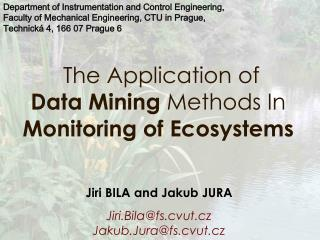Download PresentationThe Application o f Data Mining Methods In Monitoring o f EcosystemsThe Application o f Data Mining Methods In Monitoring o f Ecosystems - PowerPoint PPT Presentation

Download PresentationThe Application o f Data Mining Methods In Monitoring o f Ecosystems
An Image/Link below is provided (as is) to download presentation

Download Policy: Content on the Website is provided to you AS IS for your information and personal use and may not be sold / licensed / shared on other websites without getting consent from its author. While downloading, if for some reason you are not able to download a presentation, the publisher may have deleted the file from their server.

- - - - - - - - - - - - - - - - - - - - - - - - - - - E N D - - - - - - - - - - - - - - - - - - - - - - - - - - -
Presentation Transcript

1. Department of Instrumentation and Control Engineering, Faculty of Mechanical Engineering, CTU in Prague, Technická 4, 166 07 Prague 6 The Application of Data Mining Methods In Monitoring of Ecosystems Jiri BILAand Jakub JURA Jiri.Bila@fs.cvut.cz Jakub.Jura@fs.cvut.cz

2. Monitoring of Ecosystems • 11 Measuring Stations • 13 variables • Sampling period 6 minutes

3. Database system for monitoring

4. Data Mining • Knowledge discovery in data bases is “the nontrivial process of identifying valid, novel, potentially useful, and ultimately understandable patterns in data”Fayyad (1996).

5. Used Data MiningMethods • Conceptual Lattice • Rough Sets

6. Conceptual Lattice • Data Mining Context • C = (O, I, R) • O is a set of an objects x • I is a set of an items (attributes)y • R is a binary relation R  O I

7. Conceptual Lattice • Conceptual Lattice L • Derived from Data Mining ContextC • X = xOyY, x R y  • Y = yIxX, x R y  • X is the largest set of an objects X  O • Y is the largest set of an items Y  I

8. Conceptual Lattice • Hasse diagram • The Hasse diagram is constructed by use the partial arrangement "<„. • Edge from the H1 to H2 exist if H1 < H2 and none of element of H3 fulfil condition H1 < H3 < H2. • H1 is an antecedent of element H2 (H2 is the descendant of the element H1). • A pair of X, Y represents a node in Hasse diagram.

9. Transformace datab8ze • Hasse diagram • The Hasse diagram is constructed by use the partial arrangement "<„. • Edge from the H1 to H2 exist if H1 < H2 and none of element of H3 fulfil condition H1 < H3 < H2. • H1 is an antecedent of element H2 (H2 is the descendant of the element H1). • A pair of X, Y represents a node in Hasse diagram.

10. Conceptual Lattice - Example • C = (A0, A1, A2, A3, A4,3, 4, 7, 8, 9, R) • Where: • C … context of data mining • A0, A1, A2, A3, A4… Monitoring Classes • 3, 4, 7, 8, 9 … Situations • R … relation which is represented in the table MG

11. Monitoring Classes Situations Conceptual Lattice Table MG which represents relation R.

12. Conceptual LatticeHass diagram

13. Conceptual Lattice • Guarantee of the rule’sreliabilityand validity. • Support • supp(Ai, S) = ((s  S  Ict(s, Ai))/ ((S )) • Supp (Ai  Aj, S) = supp(Ai  Aj, S ) • Confidence • Conf (Ai  Aj, S) = Supp (Ai  Aj, S) / supp(Ai)

14. Rough Sets • Relation of indiscernibility • x1, x2 U, • (x1 RE(A) x2 )) ⇔(g(x1, ai) = g(x2, ai)) • Where: • U … universe of elements. • A … set of attributes • Vai …sets of values • g: U x A → V

15. Rough Sets • Which of these elements of universe U and with what certainty approach subset of X ⊂U, in that we are interested ? • Lower Approximation • Upper Approximation • Border set

16. Rough Sets • Lower Approximation • The Lower Approximation(positive area PosiRE(X) )is a set of objects which certain belong to a subset. • PosiRE(X) = ∪{ Y Ⅰ(Y ∈(U/RE)) AND (Y⊆ X)

17. Rough Sets • Upper Approximation • The set of elements from the U, which may (possibly) belongs to X. • PossRE(X) = ∪{YⅠ (Y ∈ U/RE) AND (Y∩ X ≠∅) }

18. Rough Sets • Boundary region • Difference between the upper and lower approximation X. • BoundRE(X) = PossRE(X) - PosiRE(X)

19. Rough Sets • Rough Set • Rough set is a subset X of universe U and this subset is defined using the upper and lower approximation (PossRE(X), PosiRE (X)) and for which: • BoundRE(X) ∅

20. Rough Sets • Rough accuracy of aproximation. • RE(X) = card (PosiRE(X)) / card (PossRE(X))

21. Conclusion • The paper proposed application of two data mining methods. Fragments of a monitoring system database have been used for the data support. The paper emphasises that the use of the original database content is not direct and it is necessary to transform it into forms utilisable by the selected data mining methods. The success of data mining process then strongly depends also on the definition of the monitoring classes and the “operation" situations (formulated by experts).